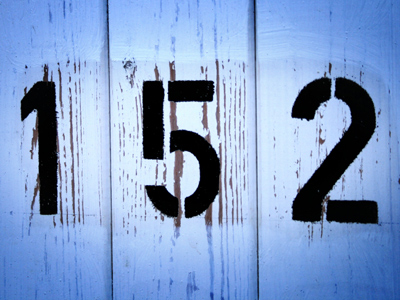152 is the next number of the sequence 112, 122, 132, 142.

# Number Sequences (Year 3)

In KS2 Maths, we explore number sequences. It starts in Year Three with simple patterns, like going up by 2, 3, 10, or 100. We also spot odd and even number patterns.

Understanding number sequences is like finding hidden patterns in numbers. Look at 1, 2, 3, 4, 5 – they go up by 1 each time. The pattern! So, if we add 1 to 5, we get 6. Can you spot patterns too?

How good are you at finding patterns in number sequences? Test your skills in this quiz for 7-8 year olds!

1.
What are the next two numbers of the sequence? 3, 6, 9, 12
15, 18
13, 14
15, 17
15, 20
The numbers are increasing in 3s
2.
If you add two even numbers together the answer is always what?
An odd number
An even number
A number ending in 2
A number ending in 0
2 even numbers always make an even number but an even with an odd will make an odd number
3.
If you add two odd numbers together the answer is always what?
An odd number
An even number
A number ending in 5
A number ending in 2
Even + even = even
Even + odd = odd
Odd + even = odd
Odd + odd = even
4.
What is the next number of the sequence? 20, 120, 220, 320
300
350
400
420
The numbers are increasing by 100
5.
Can you find the rule of this sequence? 3, 13, 23, 33
The numbers increase in 2s
The numbers increase in 3s
The numbers increase in 5s
The numbers increase in 10s
Each number (except the first) is 10 higher than the one before it
6.
Which sequence is odd?
4, 6, 8, 10, 12
30, 32, 34, 36, 38
17, 21, 25, 29, 33
12, 20, 28, 36, 44
All the numbers end in 1, 3, 5, 7 or 9 so it must be odd
7.
How do we know a number is odd?
It ends in 6
It ends in 0, 2, 4, 6 or 8
It ends in 1, 3, 5, 7 or 9
It ends in 2 or 4
If odd numbers are divided by 2 there will be a remainder or fraction left over
8.
How do we know a number is even?
It ends in 0, 2, 4, 6 or 8
It ends in 1, 3, 5, 7 or 9
It ends in 5
It ends in 9
All the even numbers can be divided by 2 and give a whole number
9.
Which is the next even number after 148?
158
150
160
152
148 + 2 = 150
10.
Can you find the rule of this sequence? 5, 10, 15, 20, 25, 30
The numbers increase in 2s
The numbers increase in 3s
The numbers increase in 5s
The numbers increase in 10s
This sequence is the 5 times table
You can find more about this topic by visiting BBC Bitesize - What is a number sequence?

Author:  Amanda Swift